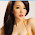NEMA 17 Stepper Motor Control using L298N Arduino tutorial

#include <Stepper.h>

const int stepsPerRevolution = 200;  // change this to fit the number of steps per revolution

// initialize the stepper library on pins 8 through 11:
Stepper myStepper(stepsPerRevolution, 8, 9, 10, 11);

int stepCount = 0;         // number of steps the motor has taken
int dirStep = 1;
void setup() {
// initialize the serial port:
Serial.begin(9600);
}

void loop() {
// step one step:
myStepper.step(dirStep);
Serial.print("steps:");
Serial.println(stepCount);
stepCount++;

if( stepCount > 500){
stepCount = 0;
if( dirStep == 1) dirStep = -1;
else dirStep = 1;
}

delayMicroseconds(50);
}

ความคิดเห็น

1.2.